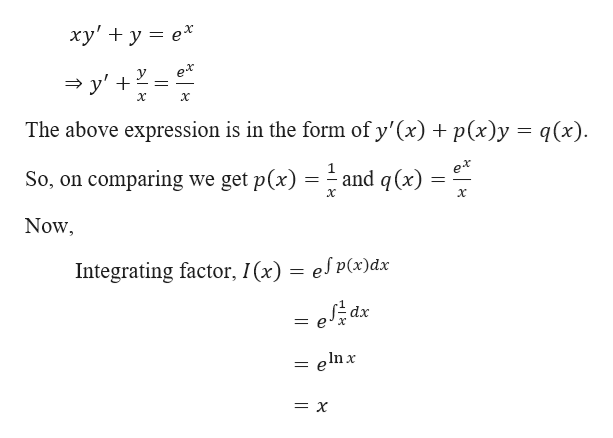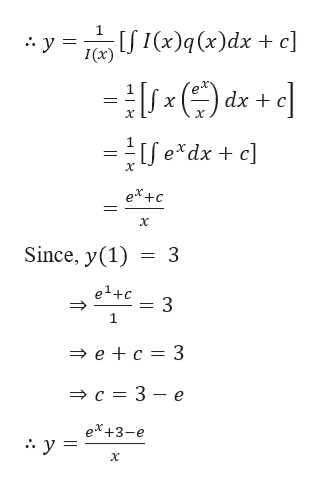# solve using the Initial Value Problem. xy' + y = ex ,       y(1) = 3

Question
18 views

solve using the Initial Value Problem.

xy' + y = ex ,       y(1) = 3

check_circle

Step 1

Given,

Step 2

Now,help_outlineImage Transcriptioncloseху' + у %3D е* ex у — у' + х х The above expression is in the form of y'(x) p(x)y = q(x) So, on comparing we get p(x) ex and q(x) х Now Integrating factor, I(x) = es P(x)dx = e#dx enx = X fullscreen
Step 3help_outlineImage Transcriptionclosey TeIdx . + c] [/ 1(х)q(x)dx + c] 1(х) (dx х х =Se*dx + c] ex+c х Since, y(1) 3 e+c 3 1 ec 3 — с %3D 3 — е eX+3-e :. у- х fullscreen

### Want to see the full answer?

See Solution

#### Want to see this answer and more?

Solutions are written by subject experts who are available 24/7. Questions are typically answered within 1 hour.*

See Solution
*Response times may vary by subject and question.
Tagged in

### Differential Equations